Function Repository Resource:

# HeegnerPrime

Convert integer tuples into Heegner field primes when applicable

Contributed by: Ed Pegg Jr
 ResourceFunction["HeegnerPrime"][{a,b},h] converts tuple {a,b} into a prime number for field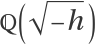when applicable.

## Details

The Heegner numbers are 1, 2, 3, 7, 11, 19, 43, 67 and 163.
Numbers within a Heegner field such as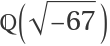have a unique factorization.

## Examples

### Basic Examples (3)

Show the Heegner primes generated by tuple {1,2}:

 In:=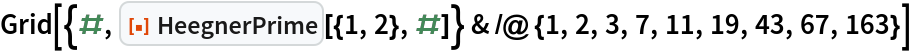Out=Tuples with a common divisor do not have corresponding Heegner primes:

 In:=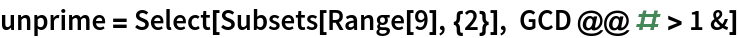Out=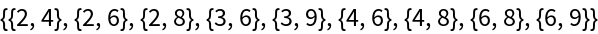Applying HeegnerPrime to these tuples gives Null:

 In:=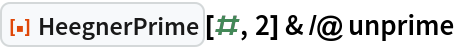Out=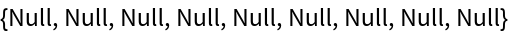Generate some Eisenstein primes from the field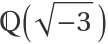:

 In:=Out=Show these in the complex plane:

 In:=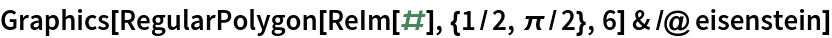Out=### Neat Examples (3)

The tuple {19,30} generates all Heegner primes, except the corresponding Gaussian prime:

 In:=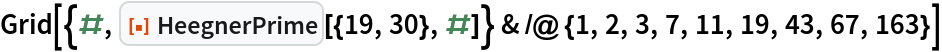Out=Generate some Gaussian primes from the field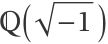:

 In:=Out=Show these in the complex plane:

 In:=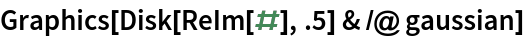Out=Show some Kleinian primes from the field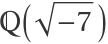:

 In:=Out=## Version History

• 1.0.0 – 02 March 2021